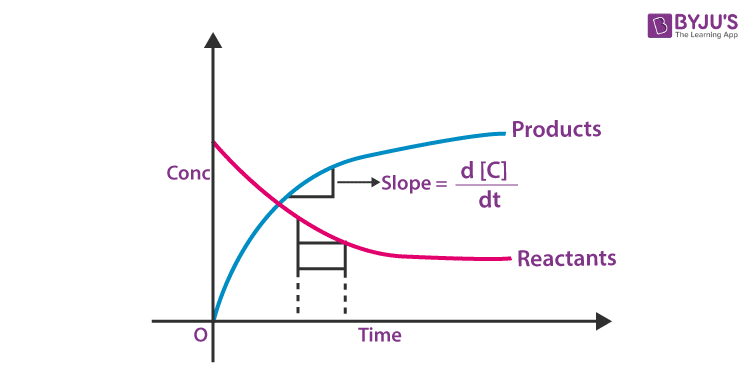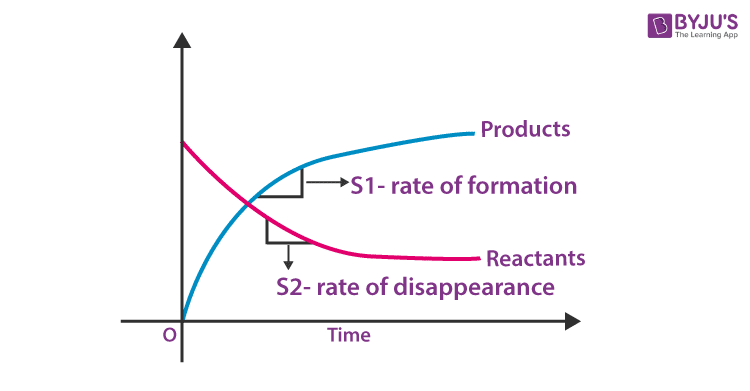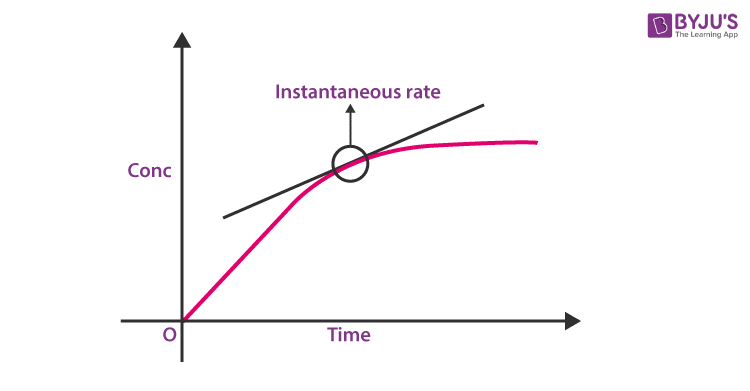# Chemical Kinetics

Chemical kinetics is an important topic in Physical Chemistry and basically deals with helping students understand the different aspects of a chemical reaction. More specifically, the term ‘kinetics’ deals with the rate of change of some quantity. For example, the rate of change of displacement is given as velocity. Likewise, acceleration is the rate of change of velocity.

Usually, based on this rate, chemical reactions can be classified as fast (eg: Na + H2O), Moderate (Mg + H2O) and slow (esterification) reactions. In this article, we will learn more about chemical kinetics and see ways to quantify the rate of a reaction and look into various factors which affect the rate of reaction.

## What is Chemical Kinetics?

Chemical kinetics also called reaction kinetics helps us understand the rates of reactions and how it is influenced by certain conditions. It further helps to gather and analyze the information about the mechanism of the reaction and define the characteristics of a chemical reaction.

## Rate of Formations and Disappearances

In any chemical reaction, as the reaction proceeds, the amount of reactants decreases, whereas the amount of products increases. One has to understand that the rate of the overall reaction depends on the rate at which reactants are consumed or the rate at which the products are formed.

If a graph is plotted between the concentration of reactants and products and time, rate of formation of products and rate of disappearance of reactants can be easily calculated from the slope of curves for products and reactants. The overall rate of the reaction may or may not be equal to the rate of formations and disappearances.(a) Product concentration is zero at time t = 0(b) at time t = 0, both reactants and products are present.

From the graph, it is understood that the slope of the reactants curve is negative and that for product curve is positive, indicating the concentration of reactants and products, decreases and increases respectively. We will take a simple reaction as an example to illustrate how the rate of overall reactions, rate of disappearances of reactants and rate of formation of products are related.

Let us take the reaction of the formation of water.

2H2 + O2 → 2H2O

From the balanced equation, it is under that, for one mole O2 consumed, 2 moles of H2 will be consumed and 2 moles of H2O will be formed. Say, the reaction proceeds for 10 mins, taking 1 mole of H2 and O2 each in the reaction mixture.

2H2 + O2 → 2H2O

t = 0 1 1 0

t = 10 mins 1 – 0.5 1 – 0.25 0.5

Say after 10 minutes, 0.5 moles of H2 is consumed and according to stoichiometry, 0.25 moles of O2 is consumed and 0.5 moles of H2O is formed. Now let us calculate the rates for H2, O2 and H2O for the first 10 minutes.

Rate of disappearance of  H2

$$\begin{array}{l}H_{2} = \frac{0.5-1}{10}\end{array}$$

$$\begin{array}{l}H_{2} = 0.05 mol/\ min\end{array}$$

Rate of disappearance of O2

$$\begin{array}{l}O_{2} = \frac{0.75-1}{10}\end{array}$$

$$\begin{array}{l}O_{2} = 0.025 mol/\ min\end{array}$$

$$\begin{array}{l}H_{2}O = 0.05 mol/\ min\end{array}$$

Rate of formation of H2O

$$\begin{array}{l}H_{2}O = \frac{0.5-0}{10}\end{array}$$

From the above calculations, we can see that rate at which H2 is consumed is twice the rate at which O2 is consumed. So, the stoichiometry of the reaction relates, rates of formation and disappearances of different reactants and products as follows. Let,

aA + bB → cC + dD

be a reaction.

$$\begin{array}{l}r=\frac{-1}{a}\frac{\Delta \left[ A \right]}{\Delta t}=\frac{-1}{b}\frac{\Delta \left[ B \right]}{\Delta t}=\frac{1}{c}\frac{\Delta \left[ C \right]}{\Delta t}=\frac{1}{d}\frac{\Delta \left[ D \right]}{\Delta t}\end{array}$$

Where r → rate of the overall reaction,

Δ[A], Δ[B], Δ[C], Δ[D] represents a change in concentration and st → change in time.

$$\begin{array}{l}\frac{\Delta \left[ A \right]}{\Delta t}\end{array}$$
→ rate of disappearance of A

$$\begin{array}{l}\frac{\Delta \left[ B \right]}{\Delta t}\end{array}$$
→ rate of disappearance of B

$$\begin{array}{l}\frac{\Delta \left[ C \right]}{\Delta t}\end{array}$$
→ rate of formation of C

$$\begin{array}{l}\frac{\Delta \left[ D \right]}{\Delta t}\end{array}$$
= rate of formation of D

Therefore,

$$\begin{array}{l}\text{rate of overall reaction}\,\text{=}\frac{\text{rate of formation/disappearance of any one component}}{\text{stoichiometric coefficient of that component}}\end{array}$$

## Average and Instantaneous Rate

The rate of reaction can be classified into average and instantaneous rate depending on the amount of time period. If the time period taken is finite, then it’s called average rate and is represented as,

$$\begin{array}{l}r\,\,\text{avg=}\frac{\Delta [C]}{\Delta t}\end{array}$$

Δl → change in concentration

Δt → change in time

r avg → average rate

Average rate doesn’t give exact information in most cases about the completion of the reaction.

For example, let us consider the hydrolysis of esters to form acid and alcohol.

$$\begin{array}{l}RCOOR’\overset{H_{2}O}{\rightarrow}RCOOH+R’OH\end{array}$$

Say at time t = 0, there was 1M solution of ester which becomes 0.5M in 30 mins. So it is logical for us to assume, that the reaction will be 100% completed in 1 hour. But in reality, the reaction takes more than 3 hours to reach completion. So, for getting a broader insight into the time taken for completion and other purposes, “Instantaneous rate is used, which is represented as,

$$\begin{array}{l}r_{inst} = \underset{\Delta t\to 0}{\mathop{\lim }}\,\frac{\Delta [C]}{\Delta t}=\frac{d[C]}{dt}\end{array}$$

From above it’s understood that, the time period taken is almost zero say from t = 0 to t = 0.0000 …1 second. This eventually comes out to be the differential of change in concentration wrt time.

For all practical purposes, the instantaneous rate is used which can be calculated from the concentration is time graph by finding a tangent at a point.Unit of rate is Moll-1 s-1 because it is concentration/time and concentration is expressed in terms of Molarity/mol l-1) it can also be Nm-2/s if the active mass is used in terms of partial pressures. Depending on other units of time it can also be mol l-1 min-1 or mol l-1 hour-1 etc.

## Factors Affecting The Reaction Rate

The rate of a reaction can be altered if any of the following parameters are changed.

### Concentration of Reactants

According to collision theory, which is discussed later, reactant molecules collide with each other to form products. If the concentration of reactants is increased, the number of colliding particles will increase thereby, increasing the rate of reaction.

### Nature of the Reactants

The reaction rate also depends on the types of substances that are reacting. If we consider acid/base reactions, salt formation and ion exchange, they are mostly fast reactions. During the formation of a covalent bond between the molecules that results in the formation of larger molecules the reaction that takes place is usually slower. Furthermore, the nature and strength of bonds in reactant molecules significantly affect the rate of their transformation into products.

### Physical State of Reactants

The physical state of a reactant whether it is solid, liquid, or gas can greatly affect the rate of change. To discuss it further, if reactants are in the same phase let’s say they are in an aqueous solution, here the thermal motion will bring them together. If they are in different phases then the reaction will be limited to the interface between the reactants.  The reaction mainly occurs only at their area of contact; in the case of a liquid and a gas, at the surface of the liquid.

### Surface Area of Reactants

If we take two solids, in this case, the particles that are at the surface will take part in the reaction. Likewise, if we were to crush a solid into smaller parts more particles will be present at the surface. What it means is that the frequency of collisions between these and reactant particles will most likely increase. As a result, the reaction will occur more rapidly.

When two or more reactants are in the same phase of fluid, their particles collide more often than when either or both are in the solid phase or when they are in a heterogeneous mixture. In a heterogeneous medium, the collision between the particles occurs at an interface between phases. Compared to the homogeneous case, the number of collisions between reactants per unit time is significantly reduced, and so is the reaction rate.

### Temperature

If the temperature is increased, the number of collisions between reactant molecules per second (frequency of collision). Increases, thereby increasing the rate of the reaction. But depending on whether the reaction is endothermic or exothermic increase in temperature increases the rate of forward or backward reactions respectively.

In a system where more than one reaction is possible, the same reactants can produce different products under different temperature conditions.

At 100 0C in the presence of dilute sulphuric acid, diethyl ether is formed from ethanol.

2CH3CH2OH → CH3CH2OCH2CH3+H2O

At 180 0C in the presence of dilute sulphuric acid, ethylene is the major product.

CH3CH2OH → C2H4+H2O

### Effect Of Solvent

The nature of the solvent also depends on the reaction rate of the solute particles.

Example:

When sodium acetates react with methyl iodide it gives methyl acetate and sodium iodide.

CH3CO2Na(sol)+CH3I(liq)CH3CO2CH3(sol)+NaI(sol)

The above reaction occurs more faster in organic solvents such as DMF (dimethylformamide) than in CH3OH (methanol), because methanol is able to form a hydrogen bond with CH3CO2 – but DMF is not possible.

### Catalyst

Catalysts alter the rate of the reaction by changing the reaction mechanism. There are two types of catalysts namely, promoters and poisons which increase and decrease the rate of reactions respectively.

For all the above factors, quantification is done in the following sections. We will try to establish a mathematical relationship between the above parameters and the rate.

Problem 1: In the reaction N2 + 3H2 → 2NH3, it is found that the rate of disappearance of N2 is 0.03 mol l-1 s-1. Calculate, the rate of disappearance of H2, rate of formation of NH3 and rate of the overall reaction.

Solution:

N2 + 3H2 → 2NH3

The rates can be connected as,

$$\begin{array}{l}r=\frac{-d\left[ {{N}_{2}} \right]}{dt}=\frac{-1}{3}\frac{d\left[ {{H}_{2}} \right]}{dt}=\frac{1}{2}\frac{d\left[ N{{H}_{3}} \right]}{dt}\end{array}$$

Given:

$$\begin{array}{l}\frac{d\left[ {{N}_{2}} \right]}{dt}=0.03mol\,{{l}^{-1}}{{s}^{-1}}\end{array}$$

Therefore, overall rate (r) = 0.03 mol l-1 s-1

Rate of disappearance of H2

$$\begin{array}{l}\left( \frac{d\left[ {{H}_{2}} \right]}{dt}=\frac{3d\left[ {{N}_{2}} \right]}{dt} \right)=0.09\,mol\,{{l}^{-1}}{{s}^{-1}}\end{array}$$

Rate of formation of

$$\begin{array}{l}N{{H}_{3}}\frac{d\left[ N{{H}_{3}} \right]}{dt}=\frac{2d\left[ {{N}_{2}} \right]}{dt}\end{array}$$
$$\begin{array}{l}N{{H}_{3}}\frac{d\left[ N{{H}_{3}} \right]}{dt}=\frac{2d\left[ {{N}_{2}} \right]}{dt}=0.06\,mol\,{{l}^{-1}}{{s}^{-1}}\end{array}$$

### Chemical Kinetics Rapid Revision### Chemical Kinetics – Top 15 JEE Mains Important and Expected Questions### Solved Questions

1. What is the rate of reaction?

Solution:

The rate of the reactions is defined as the rate at which the concentration is changing or the ratio of change in concentration and change in time

$$\begin{array}{l}r=\frac{\Delta \left[ C \right]}{\Delta t}\end{array}$$

r → rate, Δ[C] → change in concentration

Δt → change in time.

2. What is the relation between the rate of the overall reaction, rate of formation of products and rate of disappearance of reactants?

Solution:

Let aA + bB → cC + dD be a reaction, then

$$\begin{array}{l}r=\frac{-1}{a}\frac{d\left[ A \right]}{dt}=\frac{-1}{b}\frac{d\left[ B \right]}{dt}=\frac{+1}{c}\frac{d\left[ C \right]}{dt}=\frac{+1}{d}\frac{d\left[ D \right]}{dt}\end{array}$$

Where r → rate of the overall reaction

$$\begin{array}{l}\frac{-d\left[ A \right]}{dt}\to\end{array}$$
rate of disappearance of A

$$\begin{array}{l}\frac{-d\left[ B \right]}{dt}\to\end{array}$$
rate of disappearance of B

$$\begin{array}{l}\frac{-d\left[ D \right]}{dt}\to\end{array}$$
rate of formation of D

$$\begin{array}{l}\frac{+d\left[ C \right]}{dt}\to\end{array}$$
rate of formation of C

3. What are average and instantaneous rates?

Solution:

The average rate is calculated by taking a finite time period whereas the instantaneous rate is calculated for a time period that is almost tending to zero. Graphically, the slope of the graph between concentration vs time gives the average rate and tangent of a point gives instantaneous rate.

$$\begin{array}{l}{{r}_{avg}}=\frac{\Delta \left[ C \right]}{\Delta t}\,\,\,{{r}_{inst}}=\frac{d\left[ C \right]}{dt}\,\,or\,\,\underset{\Delta t\to 0}{\mathop{\lim }}\,\frac{\Delta \left[ C \right]}{\Delta t}\end{array}$$

4. Calculate the rate of disappearance of N2 in the following reactions.

N2(g) + 3H2(g) → 2NH3(g)

Given: The rate of disappearance of hydrogen gas is 0.74M/sec.

Solution:

The rate of disappearance of N2 is 1/3 the rate of disappearance of hydrogen gas

0.74 × (1/3) = 0.247 M/s.

1. What is the difference between the chemical kinetics of the reaction and chemical balancing of the equation?